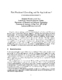## Fast Fractional Cascading and Its Applications##### Files
CS-TR-4502.ps(327.93 KB)
Using the notions of Q-heaps and fusion trees developed by Fredman and Willard, we develop a faster version of the fractional cascading technique while maintaining the linear space structure. The new version enables sublogarithmic iterative search in the case when we have a search tree and the degree of each node is bounded by $O(\log^{\epsilon}n)$, for some constant $\epsilon >0$, where $n$ is the total size of all the lists stored in the tree. The fast fractional cascading technique is used in combination with other techniques to derive sublogarithmic time algorithms for the geometric retrieval problems: orthogonal segment intersection and rectangular point enclosure. The new algorithms use $O(n)$ space and achieve a query time of $O(\log n/\log\log n + f)$, where $f$ is the number of objects satisfying the query. All our algorithms assume the version of the RAM model used by Fredman and Willard. (UMIACS-TR-2003-71)